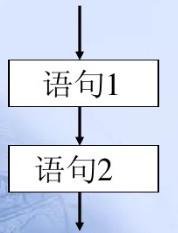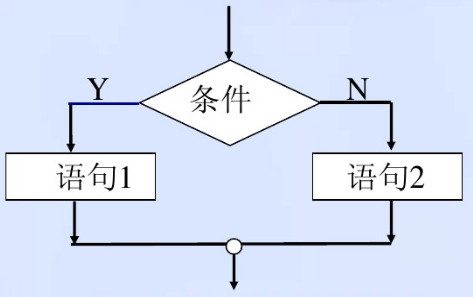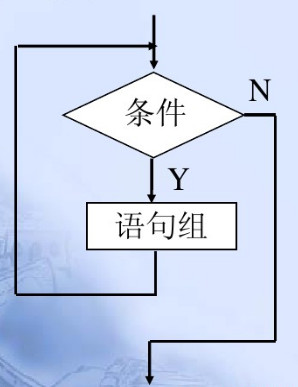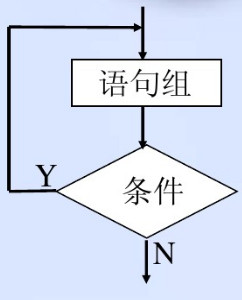• vb 循环结构设计 含有例题 ppt文件 上课用的课件
• VB循环结构

2012-11-03 01:39:15
• VB三种基本结构

万次阅读 热门讨论 2017-09-05 07:42:37
VB中最经典的东西是什么？没错就是结构，选择、顺序、循环

VB中最经典的东西是什么？没错就是三种基本结构，顺序结构选择结构、、循环结构。

1、顺序结构

程序代码按照重上到下的顺序一行一行的执行，执行过程找那个没有分支，没有重复。'输入a b
'交换a b 的值
Dim a As Integer
Dim b As Integer
a = Val(InputBox("a"))
b = Val(InputBox("b"))
c = a
a = b
b = c
MsgBox ("交换后a=" & a & "b=" & b)

2、选择结构

根据不同的条件判断来决定程序执行走向。a)单分支结构

'当a大于1时  显示a
If a > 1 Then
Print a
End If

b)双分支结构

'比较X,Y两个值中较大的一个

If x > Y Then
Print x
Else
Print Y
End If

c)多分支结构

'按成绩等级分类
If x >= 90 Then
Print "优秀"
ElseIf x >= 80 Then
Print "良好"
ElseIf x >= 70 Then
Print ""
ElseIf x >= 60 Then
Print ""
Else
Print ""
End If

d)情况语句

3、循环结构

根据条件判断某些语句可能需要执行0次1次或多次。

1)当型循环'循环次数
Dim I As Long, S As Long
For I = 1 To 100
S = S + I
Next
MsgBox "For...Next：1+2+3+...+100 = " & S

Dim I As Long, S As Long
I = 0: S = 0
Do While I < 100
I = I + 1
S = S + I
Loop
MsgBox "Do While：1+2+3+...+100 = " & S

Dim I As Long, S As Long
I = 0: S = 0
Do Until I > 100
S = S + I
I = I + 1
Loop
MsgBox "Do Until：1+2+3+...+100 = " & S

Dim I As Long, S As Long
I = 0: S = 0
While I < 100
I = I + 1
S = S + I
Wend
MsgBox "While...Wend：1+2+3+...+100 = " & S

2)直到型循环Dim I As Long, S As Long
I = 0: S = 0
Do
I = I + 1
If I > 100 Then Exit Do
S = S + I
Loop
MsgBox "Do...Loop：1+2+3+...+100 = " & S

Dim I As Long, S As Long
I = 0: S = 0
Do
I = I + 1
S = S + I
Loop While I < 100
MsgBox "Loop While：1+2+3+...+100 = " & S

Dim I As Long, S As Long
I = 0: S = 0
Do
S = S + I
I = I + 1
Loop Until I > 100
MsgBox "Loop Until：1+2+3+...+100 = " & S

展开全文• vb控制结构循环结构程序设计PPT课件.pptx
• VB的基本语法之循环结构

千次阅读 2020-05-15 17:17:44
VB班含有两种循环结构，一是计数循环，一是条件循环。其中，条件循环包括有：直到型循环和当型循环。 （一）计数循环 For-Next循环是计数循环，常用于循环次数已知的情况。 'For-Next循环结构 For 循环变量=初值...

VB的基本语法之循环结构

VB班含有两种循环结构，一种是计数循环，一种是条件循环。其中，条件循环包括有：直到型循环和当型循环。
（一）计数循环
For-Next循环是计数循环，常用于循环次数已知的情况。

'For-Next循环结构
For 循环变量=初值 To 终值 （step 步长）
语句块1Exit for）
语句块2
Next 循环变量

（二）条件循环之直到型循环
Do-Loop循环具有两种语法结构，分别是前测型和后测型循环。引用本人对于直到型循环的总结的一句话:直到型循环就是程序不断执行，直到不满足限定条件为止。

'前测型循环结构
Do 条件
语句块1
(Exit Do)
语句块2
Loop

'后测型循环结构
Do
语句块1
(Exit Do)
语句块2
Loop 条件

（三）条件循环之当型循环
While-Wend循环具有两种语法结构，分别是前测型和后测型循环。引用本人对于直到型循环的总结的一句话:当型循环就是当满足条件程序不断执行，直到不满足限定条件为止。

'前测型循环结构
whlie 条件
语句块1
(Exit Do)
语句块2
wend

'后测型循环结构
whlie
语句块1
(Exit Do)
语句块2
wend 条件

展开全文• 高中信息技术（算法与程序设计）——vb循环结构教案
• VB中几种循环结构

千次阅读 2016-04-17 16:54:27
Private Sub Command1_Click() Cls Dim n As Integer Dim s As Integer Dim a(10) As Integer n = 1 While n a(n) = Int(Rnd * 1000) n = n + 1 Wend n = 1 While n Print a(n) ...Wend
Private Sub Command1_Click()
Cls
Dim n As Integer
Dim s As Integer
Dim a(10) As Integer

n = 1
While n <= 10
a(n) = Int(Rnd * 1000)
n = n + 1
Wend
n = 1
While n <= 10
Print a(n)
n = n + 1
Wend

s = 0
n = 1
While n <= 10
s = s + a(n)
n = n + 1
Wend

Print s

End Sub

2.

Private Sub Command1_Click()
Dim i As Integer
Dim sum As Integer
Dim a As Integer

Do
Randomize
a = Int(Rnd * 1000)
sum = sum + a
i = i + 1
Loop Until i > 10
MsgBox "10个随机数得和:" & sum

End Sub

3.

Private Sub Command1_Click()
Dim i As Integer
Dim sum As Integer
Dim a As Integer

Do
Randomize
a = Int(Rnd * 1000)
sum = sum + a
i = i + 1
Loop while i <= 10
MsgBox "10个随机数得和:" & sum

End Sub

4.

Private Sub Command1_Click()
Dim i As Integer
Dim sum As Integer
Dim a As Integer

i = 1
Do While i <= 10
Randomize
a = Int(Rnd * 1000)
sum = sum + a
i = i + 1
Loop
MsgBox "10个随机数得和:" & sum

End Sub

5.

Private Sub Command1_Click()
Dim i As Integer
Dim sum As Integer
Dim a As Integer

i = 1
Do Until i > 10
Randomize
a = Int(Rnd * 1000)
sum = sum + a
i = i + 1
Loop
MsgBox "10个随机数得和:" & sum

End Sub

6.

Private Sub Command1_Click()
Cls
Print f(10)

End Sub
Private Function f(x As Integer) As Long
If x = 1 Then
f = x
Else
f = x + f(x - 1)
End If

End Function

7.

Private Sub Command1_Click()
Dim sum As Integer
Dim i As Integer
Dim a As Integer
sum = 0
For i = 1 To 10
Randomize
a = Int(1000 * Rnd)
sum = sum + a
Next
MsgBox "10个随机数得和:" & sum

End Sub

8.

Private Sub Command1_Click()
Cls

Dim n As Integer
Dim s As Integer
Dim a(10) As Integer

n = 1
While n <= 10
a(n) = Int(Rnd * 1000)
n = n + 1
Wend
n = 1
While n <= 10
Print a(n)
n = n + 1
Wend

s = 0
n = 1

For Each t In a
s = s + t
Next

Print s

End Sub

展开全文• 本文档主要讲述的是Visual Basic循环结构程序设计；在指定的条件下多次重复执行一组语句。VB中提供了两类型的循环语句： 计数循环语句和条件循环语句。循环体中要有控制循环的语句，以避免出现死循环。在循环体中...
•文档
• VB语言循环结构，让你轻松尝到VB的强大之处，让你爱上VB
• VB程序设计—For循环结构

千次阅读 2020-04-20 18:52:47
for循环 1.语法结构 2.执行顺序 3.注意说明 （一）语法结构： For 循环变量=初值 to 终值【step 步长】 【循环体】 【Exit for】 Next 【循环变量】【，循环变量】 计算1累加到100的和 Dim i as integer '声明变量i...

3.注意说明

（一）语法结构：
For 循环变量=初值 to 终值【step 步长】
【循环体】
【Exit for】
Next 【循环变量】【，循环变量】

计算1累加到100的和

Dim i as integer         '声明变量i
Dim sum as long          '声明变量sum
i=1:sum=0                '将1赋值给变量i，将0赋值给变量sum
For  i=1 to 100 step 1   '循环1-100之间的数，循环完一次就将i+1
sum=i+sum        '将sum+i的值赋值给新的sum
Next i
print sum                '循环结束后输出结果sum

执行顺序

①将1赋值给i，然后判断i是否在1-100区间内
②如果在，执行循环体内的sum=sum+i
③由于step 1，所以相当于变量i=i+1
④再继续判断是否在1-100区间内
⑤一直判断到 i>100 跳出循环，输出sum

说明
1、步长：默认为1时可以省略，不可为0
2、循环次数：Int（终值-初值)/步长+1
3、For-Next循环次数可以使用嵌套循环

for循环的嵌套使用

Dim i as integer
Dim j as integer
i=1:j=1
For  i=1 to 2 step 1
print "*"
For j=1 to 2 step 1
print "#"
Next j
Next i
print sum

for嵌套的例子
九九乘法表

Dim i as Integer
Dim j as Integer
i=1:j=1
For i=1 to 9
For j=1 to i  '注意一行里面输出的列的个数不能超过行的个数；所以终值是i所以第一个循环跟第二个的循环次数相同
Print i & "*" & j & "=" & i * j;space(2);   ’在输出语句后面加分号（;）代表着 连着输出不换行
Next j
print   '把第一行循环的结果全部输出在一行上 ，再换行
Next i

展开全文• VB程序实现算法三种基本结构专题复习课前准备算法解决问题的方法和步骤三种基本结构 顺序结构 分支结构 循环结构 选择重复1基本知识点学案130520.doc基本知识点表格思考该题是什么结构2程序设计讲与练例1先后输入...
•学习课件
• 计算机软件及应用vb循环结构PPT课件.pptx
• vb循环结构程序设计实例PPT教案学习.pptx
• VB程序的基本结构――循环结构之For-Next语句》由会员分享，可在线阅读，更多相关《VB程序的基本结构――循环结构之For-Next语句(4页珍藏版)》请在人人文库网上搜索。1、课 题： 程序的基本结构循环结构之For-Next...
• 资料收集于网络如有侵权 请联系网站删除 循环结构测试题(四) 一选择题 1以下 是正确的for.next结构 A for x=1 to step 10 (B) for x=3 to 3 step -3 . . next x next x (C ) for x=1 to 10 (D) for x=3 to step 3 re...
• 使用循环结构实现次密码输入错误强制退出系统的案例循环结构
• 计算机软件及应用vb循环结构PPT学习教案.pptx
• VB程序实现算法三种基本结构课件 课前准备 算法解决问题的方法和步骤 三种基本结构 顺序结构 分支结构 循环结构 选择 重复 1基本知识点 基本知识点表格 2程序设计讲与练 例1先后输入使用r=inputbox)两个正整数xy,把...
• VB中do循环的四种格式

千次阅读 2019-09-08 17:35:44
VB中，do循环具有四格式。 格式1： do while 条件 循环体 loop 格式2： do until 条件 循环体 loop 格式3： do 循环体 loop while 条件 格式4： do 循环体 loop until 条件 下面是这4格式的4个例子，...
• 适合思考和独立循环控制语句是基本流程控制语句之一。自主学习，比较适合学习VB。提供三种不同风格的循环语句
• 课前准备 算法解决问题的方法和步骤 三种基本结构 顺序结构 分支结构 循环结构 选择 重复 1基本知识点 基本知识点表格 2程序设计讲与练 例1先后输入使用r=inputbox)两个正整数xy,把较大的数的值赋值给变量max并输出 ...
• 本文简要介绍Visual Basic 6.0与Python 3.x的循环结构以及将两者做简明对比，梳理其中的循环逻辑。一、自然简洁的表达方式我们从Python中的While语句讲起。While语句在Python中成为无限循环或条件循环，其语法很简单...
• For Each…Next语句用于逐一循环数组或集合中的每个元素。 语法格式如下： For Each <数组或集合中的元素> in <数组或集合> <循环体> [Exit For] Next <数组或集合中元素> 说明： 数组或...
• VB程序设计中的循环语句模块采用问题驱动的教学方法,通过问题引发学生对循环语句的基本概念、执行机制和控制原理进行思考,培养学生分析问题、解决问题的能力,改善教学效果,提高教学质量。...